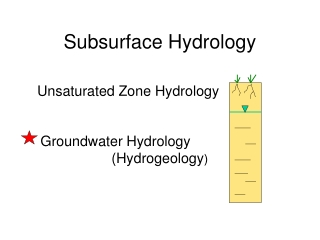DownloadDownload PresentationSubsurface Hydrology

# Subsurface Hydrology

Download Presentation## Subsurface Hydrology

- - - - - - - - - - - - - - - - - - - - - - - - - - - E N D - - - - - - - - - - - - - - - - - - - - - - - - - - -
##### Presentation Transcript

1. Subsurface Hydrology Unsaturated Zone Hydrology Groundwater Hydrology (Hydrogeology)

2. R = P - ET - RO ET ET P E RO R R / P = ? Water Table Groundwater

3. Processes we might want to model • Groundwater flow • calculate both heads and flow • Solute transport – requires information on flow (velocities) • calculate concentrations v = K I / n

4. Types of Models • Physical (e.g., sand tank) • Analog (electric analog, Hele-Shaw) • Mathematical

5. Hele-Shaw Model

6. Types of Models • Physical (e.g., sand tank) • Analog (electric analog, Hele-Shaw) • Mathematical

7. Components of a Mathematical Model • Governing Equation (Darcy’s law + water balance eqn) with head (h) as the dependent variable • Boundary Conditions • Initial conditions (for transient problems)

8. Toth Problem Groundwater divide Groundwater divide Laplace Equation Impermeable Rock 2D, steady state

9. Conceptual Model A descriptive representation of a groundwater system that incorporates an interpretation of the geological & hydrological conditions. Generally includes information about the water budget.

10. Types of Solutions of Mathematical Models • Analytical Solutions: h= f(x,y,z,t) • (example: Theis eqn.) • Numerical Solutions • Finite difference methods • Finite element methods • Analytic Element Methods (AEM)

11. Topo-Drive Finite element model of a version of the Toth Problem for regional flow in cross section.

12. Finite difference models • may be solved using: • a computer programs (e.g., a FORTRAN program) • a spreadsheet (e.g., EXCEL)

13. MODFLOW •  USGS code •  Finite Difference Model • MODFLOW 88 • MODFLOW 96 • MODFLOW 2000

14. Steps in Groundwater Modeling • 1. Model Design • Calibration (History matching) • Prediction (head)